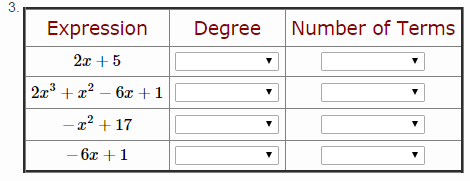## Multiple Matching with Variables

Follow
Feature idea (LEVEL 1)

I'm trying to make a question where students have to classify a given expression by its degree and the number of terms. Since it is going to be a review assignment, I want to use variables so they get a new question for each attempt.

My problem is that Buzz normally won't list potential answers more than once. Since I'm using variables however, Buzz isn't "seeing" the text pulled by the variable to recognize the words used multiple times. This causes another issue too. Say the answer "monomial" is used 3 times. Buzz will only recognize one of those as the correct answer. There is no way to know which. Thirdly, because Buzz doesn't recognized the actual text string of the answers, I can't put in detractors because they will all appear multiple times.

Is there a way for Buzz to recognize the text of the answer the variable is pulling in? If not, please consider this a feature request.

Here is a picture of the question and another with the menu up. The code of the question is pasted at the end of the post.Type: MT
Options: Inline, Multiple
Score: 8, Partial
Var: a.expression = 7,2x+5,5x^2-8,-x^2+3x-4,x^3
Var: a.terms = monomial,binomial,binomial,trinomial,monomial
Var: b.expression = 2x^3+x^2-6x+1,x,3,7x^2+5x-4,4x^3+1
Var: b.terms = polynomial,monomial,monomial,trinomial,binomial
Var: c.expression = 16,3x-10,-x^2+17,x^2-8x,-2x^3
Var: c.terms = monomial,binomial,binomial,binomial,monomial
Var: d.expression = x^4+x^2,-6x+1,x^4,3x,-3x^2-4x+11,-13x^3+12
Var: d.terms = binomial,binomial,monomial,monomial,trinomial,binomial
<table style="border-collapse: collapse;" border="3">
<tbody><tr>
<td style="padding:5px;" align="center"><span style="font-size:14pt; color: darkred;">Expression</span>
</td>
<td style="padding:5px;" align="center"><span style="font-size:14pt; color: darkred;">Degree</span>
</td>
<td style="padding:5px;" align="center"><span style="font-size:14pt; color: darkred;">Number of Terms</span>
</td>
</tr>
<tr>
</tr>
<tr>
</tr>
<tr>
</tr>
<tr>
</tr>
</tbody></table>
</div>[/HTML]
a. 1 = \$a.degree\$
b. 2 = \$a.terms\$
c. 3 = \$b.degree\$
d. 4 = \$b.terms\$
e. 5 = \$c.degree\$
f. 6 = \$c.terms\$
g. 7 = \$d.degree\$
h. 8 = \$d.terms\$
i. = monomial
j. = binomial
k. = trinomial
l. = polynomial
m. = constant
n. = linear